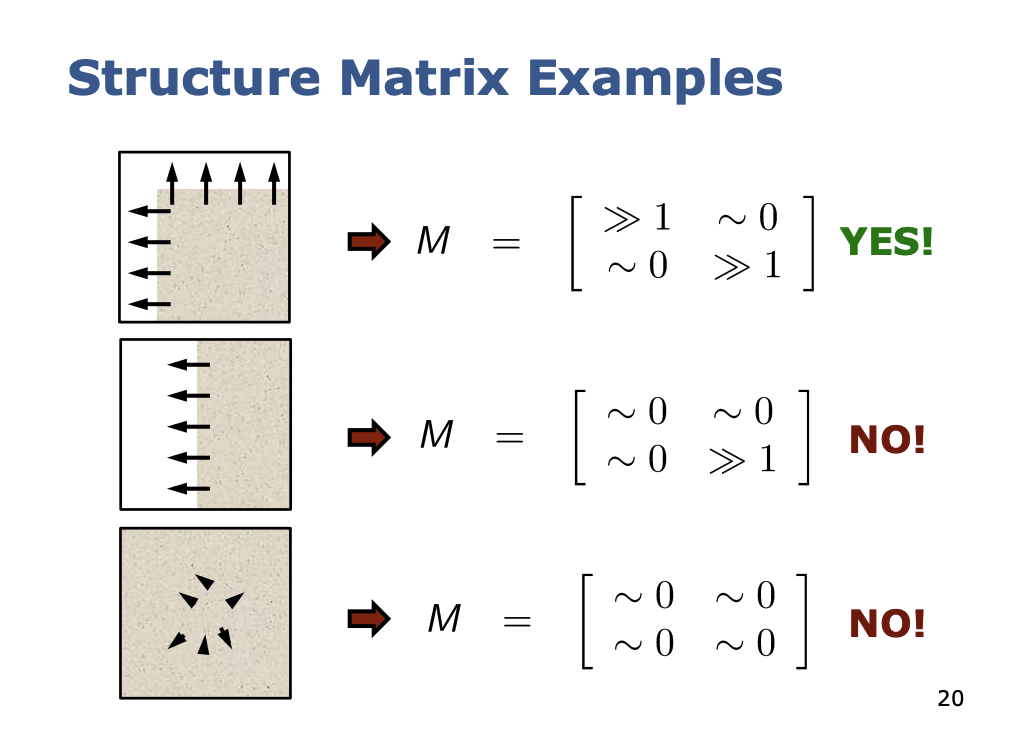Keypoint

# Structure Matrix §

Taught to me by Cyrill Stachniss.

The structure matrix is key to finding edges and corners in an image. It encodes the changes in image intensities in a local area.

• summarizes the dominant directions of the gradient around a point

The structure matrix is constructed by computing gradients (partial derivatives) of the image in both and direction

• Here, and are the gradients (jacobians) of the image at that local patch

How can we calculate these gradients? It seems super intimidating, but you can simply use a kernel!

Use these two approximations:https://www.ipb.uni-bonn.de/html/teaching/photo12-2021/2021-pho1-10-features-keypoints.pptx.pdf

Go to Keypoint to see more about the ways this structure matrix is precisely calculated.

### Derivation §

The derivation of a structure matrix comes from the idea that we need to search for intensity changes in two directions.